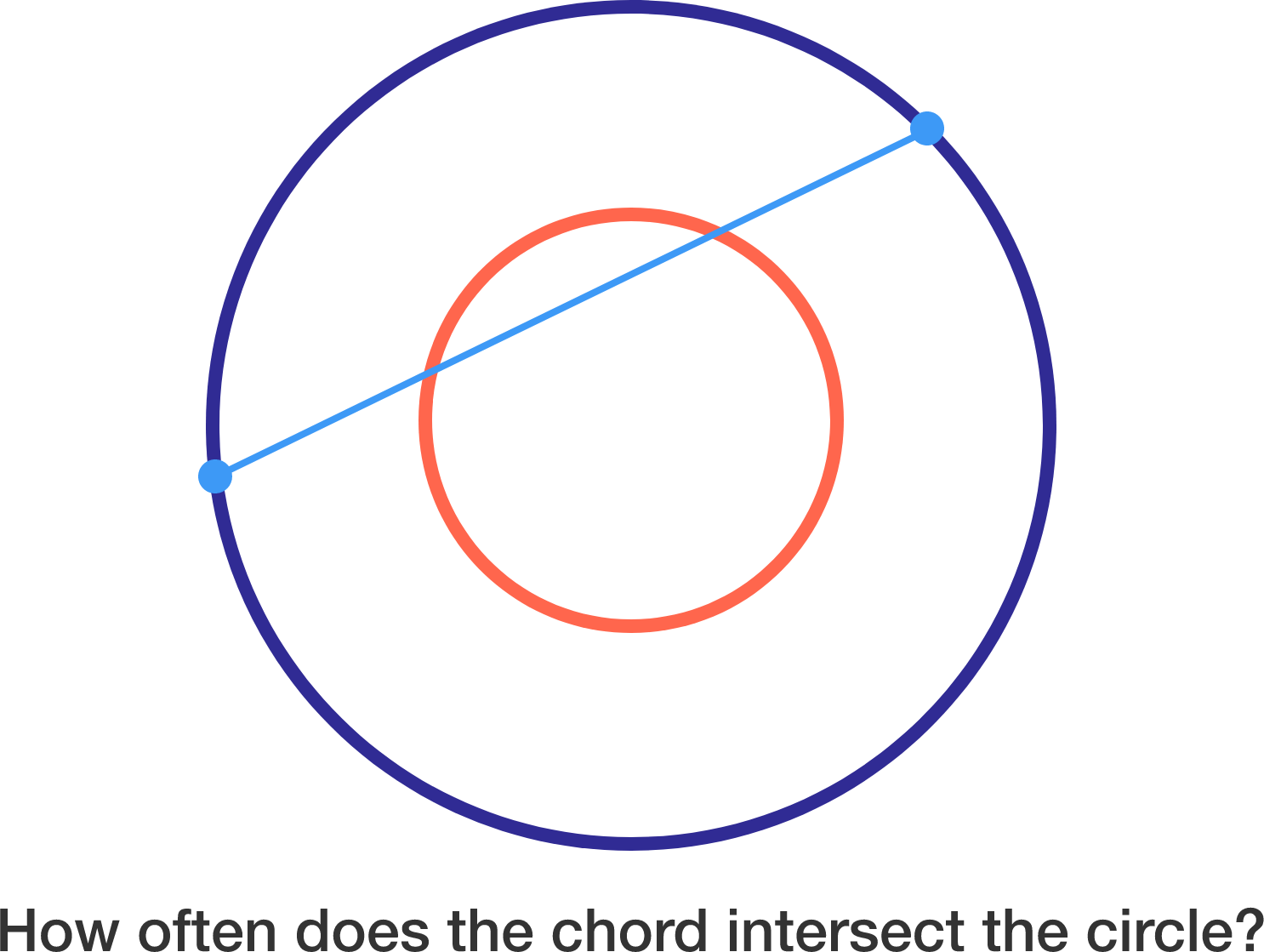# Strike a chord

Probability Level 4

Consider concentric circles with respective radii $\pi$ and $2\pi$. Choose two points on the larger circle independently and uniformly at random, and then join them to form a chord. If $P$ is the probability that this chord intersects the smaller circle at at least one point, then find $\lfloor 1000P \rfloor$.Notation: $\lfloor \cdot \rfloor$ denotes the floor function.

×